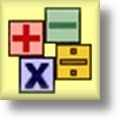Mixed Problems Worksheets

Mixed Problems Worksheets for Practice

Here is a graphic preview for all of the Mixed Problems Worksheets. You can select different variables to customize these Mixed Problems Worksheets for your needs. The Mixed Problems Worksheets are randomly created and will never repeat so you have an endless supply of quality Mixed Problems Worksheets to use in the classroom or at home. Our Mixed Problems Worksheets are free to download, easy to use, and very flexible.

These Mixed Problems Worksheets are a great resource for children in Kindergarten, 1st Grade, 2nd Grade, 3rd Grade, 4th Grade, and 5th Grade.

Click here for a Detailed Description of all the Mixed Problems Worksheets.

Quick Link for All Mixed Problems Worksheets

Click the image to be taken to that Mixed Problems Worksheet.

Single DigitAddition and SubtractionMixed Problems WorksheetsSingle Digitfor + - ×Mixed Problems Worksheets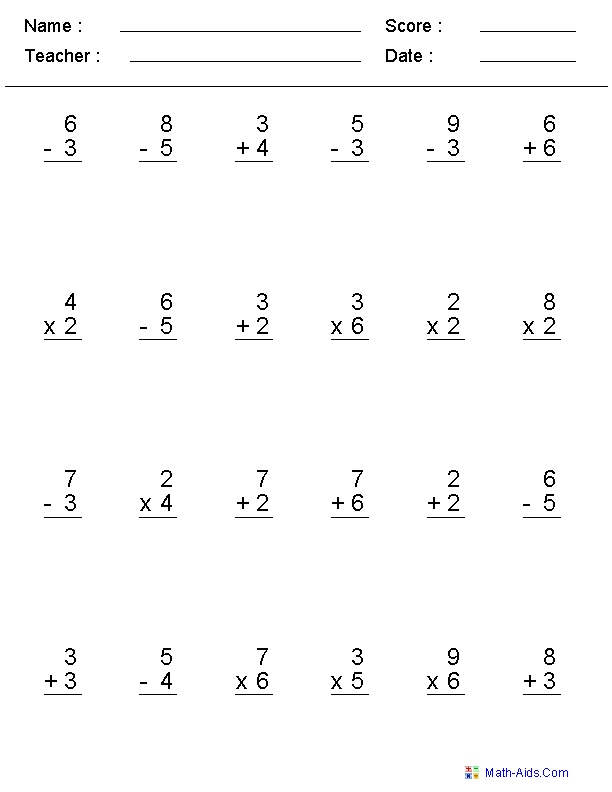Adding & SubtractingWith DotsMixed Problems Worksheets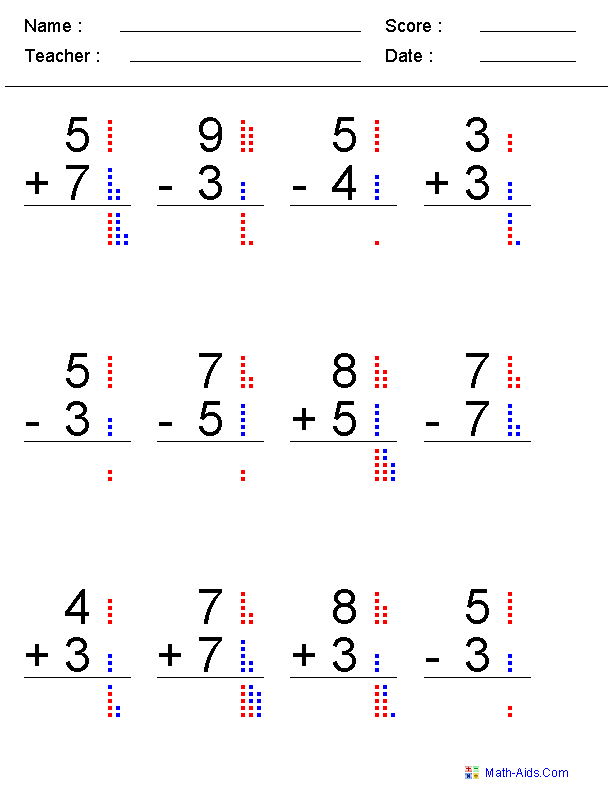1 or 2 Digitfor + - ×Mixed Problems Worksheets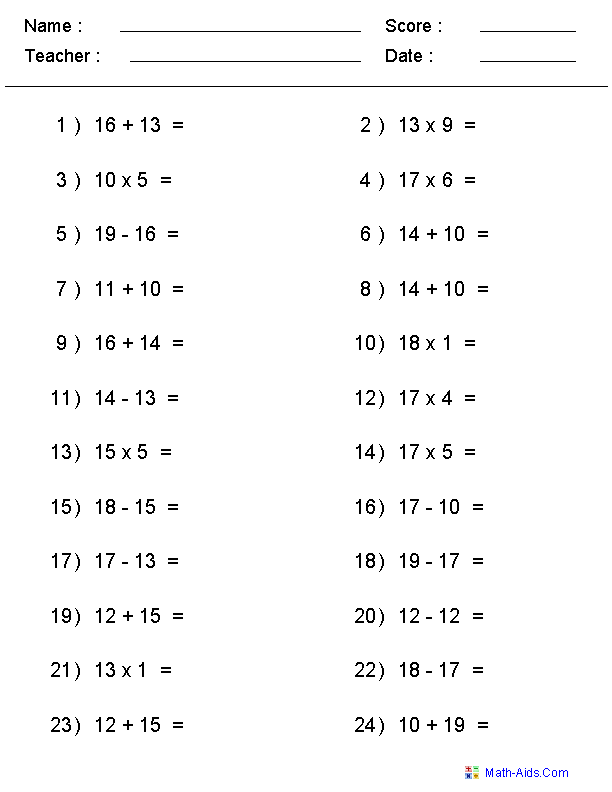Solving Equality EquationsMixed Problems Worksheets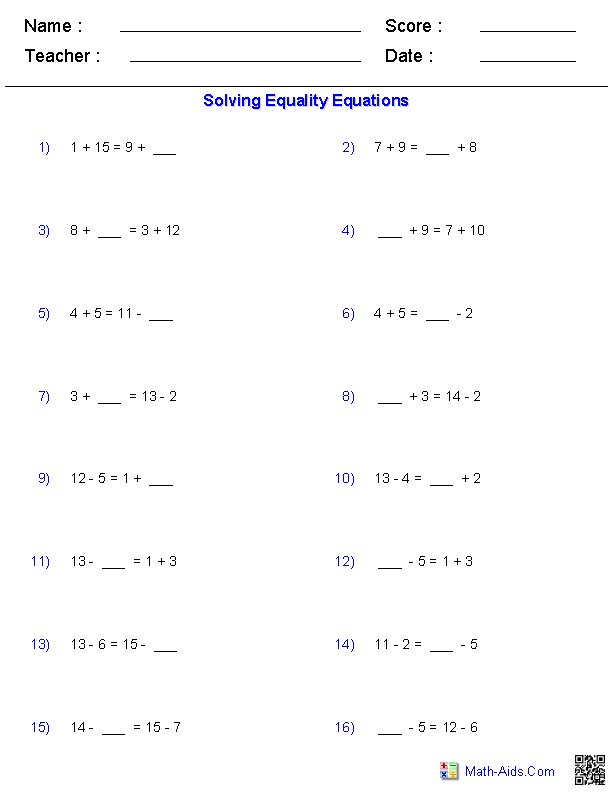1 or 2 Digit - 4 Numbersfor Addition and SubtractionMixed Problems WorksheetsAdding and Subtracting2, 3, or 4 Digit ProblemsMixed Problems Worksheets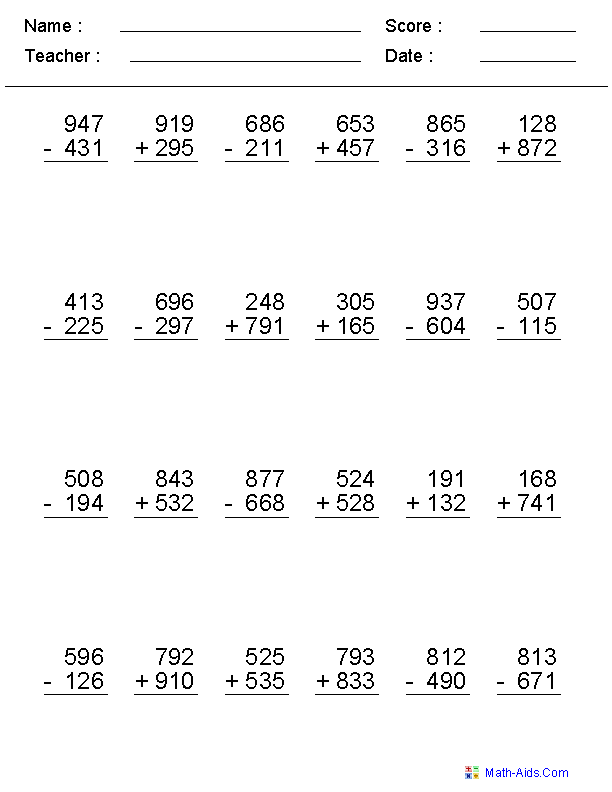Adding and Subtracting4, 5, or 6 Digit ProblemsMixed Problems WorksheetsAdding & SubtractingWith No RegroupingMixed Problems Worksheets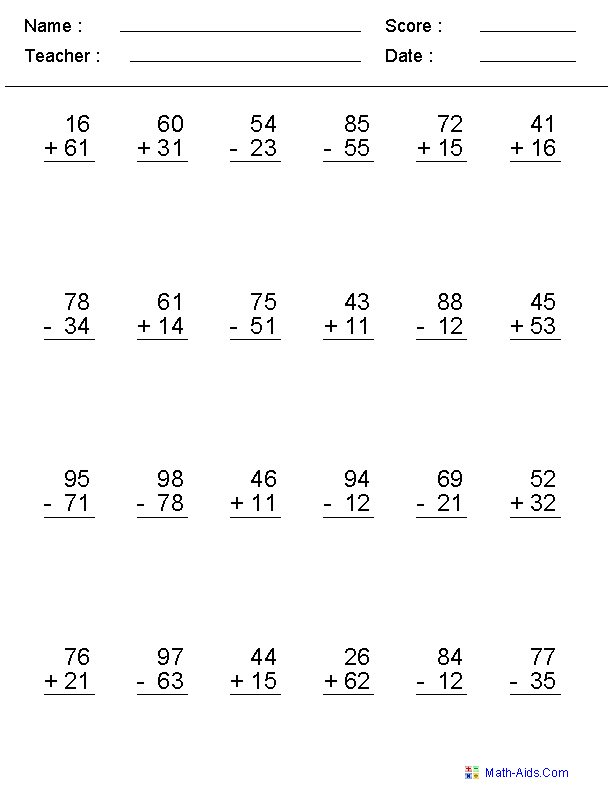Adding & SubtractingDecimal NumbersMixed Problems Worksheets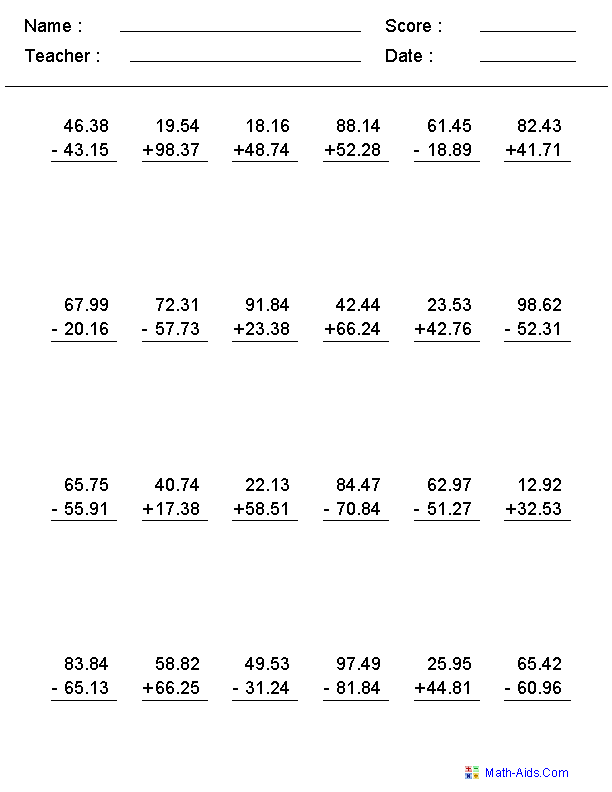Adding & SubtractingMoney NumbersMixed Problems WorksheetsNegative Numbersfor + - ×Mixed Problems Worksheets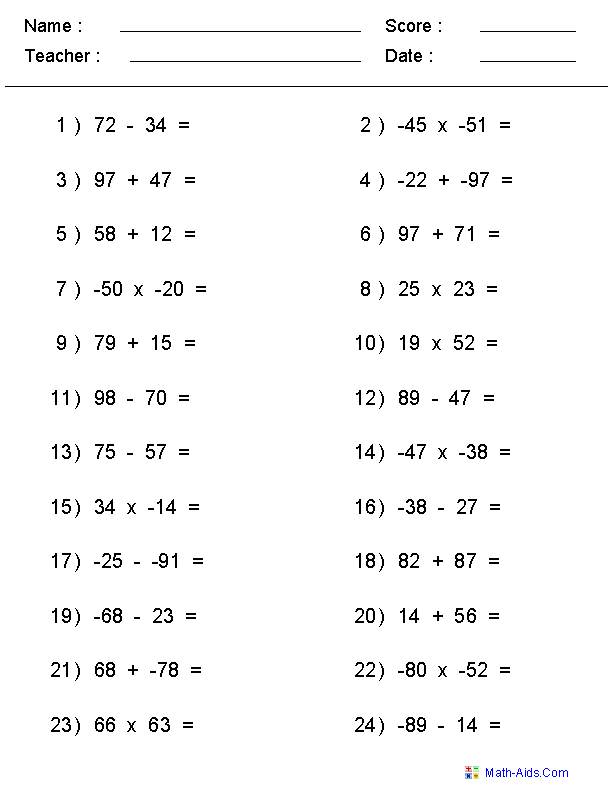Missing Numbersfor + - × ÷Mixed Problems Worksheets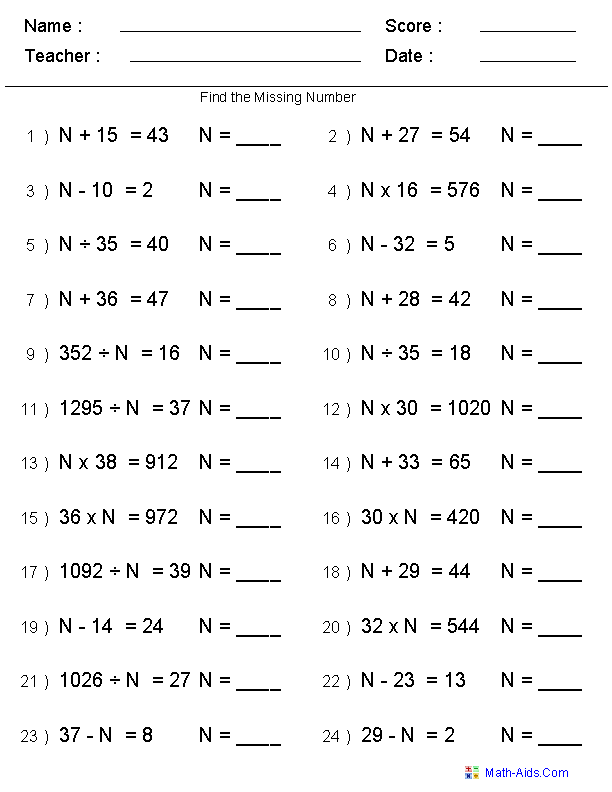1, 3, or 5 Minute Drillsfor + - × ÷Mixed Problems Worksheets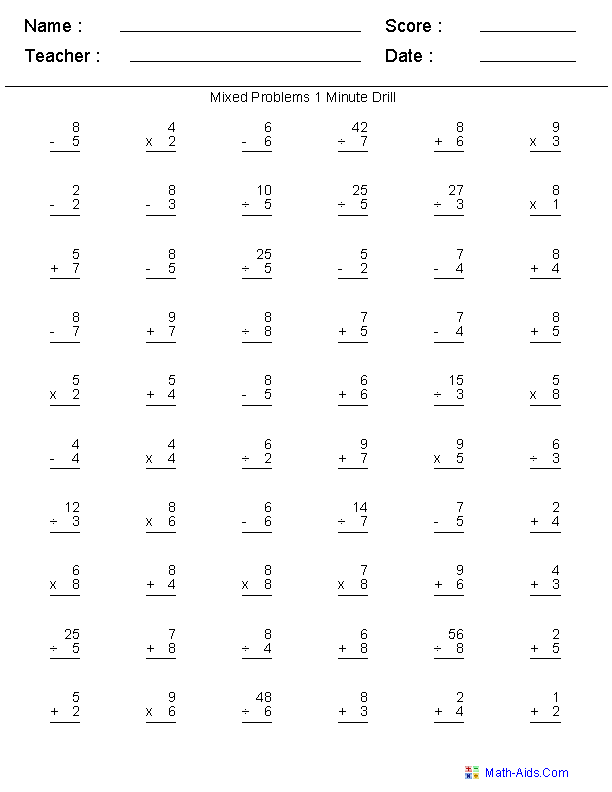Adding & SubtractingMissing DigitsMixed Problems Worksheets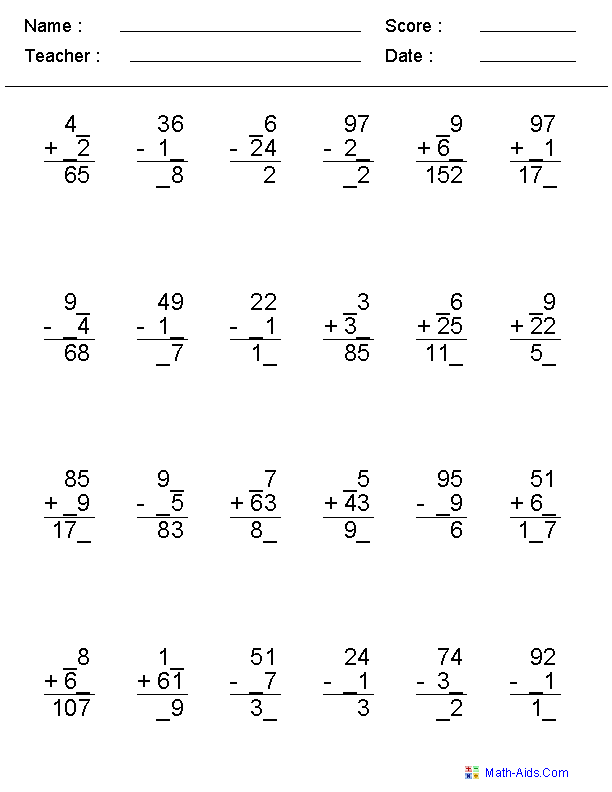Adding & SubtractingIrregular UnitsMixed Problems Worksheets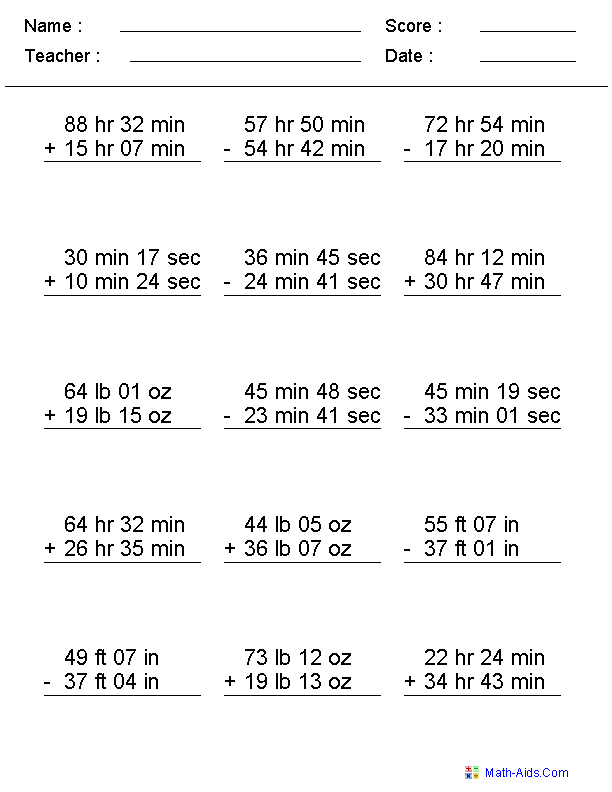Adding & SubtractingMultiplying & DividingMixed Problems WorksheetsAdding & SubtractingMultiplying & DividingTwo Fractions Worksheets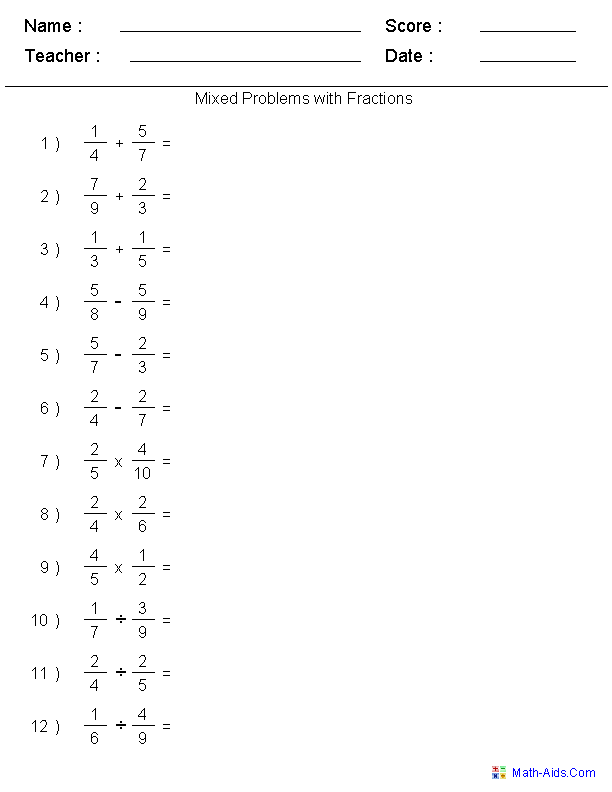Recommended Videos

Detailed Description for All Mixed Problems Worksheets

Single Digit for Addition and Subtraction
Mixed Problems Worksheets

These single digit addition and subtraction worksheets are configured for 2 numbers in a vertical problem format. The range of numbers used for each worksheet may be individually varied to generate different sets of mixed operator problems.

Single Digit for Addition, Subtraction, & Multiplication
Mixed Problems Worksheets

These single digit mixed problems worksheets are configured for 2 numbers in a vertical problem format. The range of numbers used for each worksheet may be individually varied to generate different sets of mixed operator problems.

Adding & Subtracting with Dots
Mixed Problems Worksheets

These mixed problems worksheets will produce 12 vertical problems with dots to the right of each number to aid the children with the addition or subtraction. The range of numbers used for each worksheet may be individually varied to generate different sets of problems.

Single or Multiple Digit for Addition, Subtraction, & Multiplication
Mixed Problems Worksheets

These mixed problems worksheets may be configured for either single or multiple digit horizontal problems with 2 numbers. You may select between 12 and 30 problems to be displayed on each worksheet.

Solving Equality Equations
Mixed Problems Worksheets

These mixed problems worksheets are great for testing students on solving equalities in an equation. You may select four different variations of the location for the unknown. You may select between 12, 16, and 20 problems to be displayed on each worksheet.

1 or 2 Digit - 4 Numbers for Addition and Subtraction
Mixed Problems Worksheets

These mixed problems worksheets will produce a four number addition and subtraction problem in a horizontal format with one of the numbers left out. You may select between 12 & 24 problems to be displayed on each worksheet.

Adding and Subtracting 2, 3, or 4 Digit Problems
Mixed Problems Worksheets

These mixed problems worksheets may be configured for adding and subtracting 2, 3, and 4 digit problems in a vertical format. For the subtraction problems you may select some regrouping, no regrouping, all regrouping, or subtraction across zero. You may select up to 30 mixed problems per worksheet.

Adding and Subtracting 4, 5, or 6 Digit Problems
Mixed Problems Worksheets

These mixed problems worksheets may be configured for adding and subtracting 4, 5, and 6 digit problems in a vertical format. For the subtraction problems you may select some regrouping, no regrouping, all regrouping, or subtraction across zero. You may select up to 20 mixed problems per worksheet.

Adding & Subtracting With No Regrouping
Mixed Problems Worksheets

These mixed problems worksheets are great for problems that do not require regrouping. The addition and subtraction problems may be configured with either 2 digits (plus/minus) 1 digit problems or 2 digits (plus/minus) 2 digits problems. The no regrouping option may be switched off if some regrouping is desired. The problem format is vertical and you may select up to 30 mixed problems per worksheet.

Adding & Subtracting Decimal Numbers
Mixed Problems Worksheets

These Mixed Problems Worksheets may be configured for 1, 2, and 3 Digits on the right of the decimal and up to 4 digits on the left of the decimal. You may select up to 25 Mixed Problems per worksheet.

Adding & Subtracting Money Numbers
Mixed Problems Worksheets

These Mixed Problems Worksheets may be configured for up to 4 digits in each problem. The currency symbol may be selected from Dollar, Pound, Euro, and Yen. You may select up to 25 mixed problems per worksheet.

Negative Numbers for Addition, Subtraction, & Multiplication
Mixed Problems Worksheets

These mixed problems worksheets may be configured for either single or multiple digit horizontal problems. The numbers may be selected to be positive, negative or mixed. You may vary the numbers of problems on each worksheet from 12 to 30.

Missing Numbers for Addition, Subtraction, Multiplication, and Division
Mixed Problems Worksheets

These mixed problems worksheets are a good introduction for algebra concepts. You may select various types of characters to replace the missing numbers. The formats of the problems are horizontal and the numbers range from 0 to 99. You may select up to 30 mixed problems per worksheet.

1 or 5 Minute Drills for Addition, Subtraction, Multiplication, and Division
Mixed Problems Worksheets

These mixed problems worksheets are for timed drills. They contain single digit addition, subtraction, multiplication, and division problems on one page. A student who has memorized all of these single digit problems should be able to work out these mixed problems worksheets correctly in the allowed time. You may select which operations you wish to use, and vary the number range as well.

Adding & Subtracting Missing Digits
Mixed Problems Worksheets

These mixed problems worksheets are configured for a vertical problem format. The missing digits are randomly selected to challenge the children in solving the problems. The number of digits in each problem may be varied between 2 and 4. You may select up to 30 mixed problems per worksheet.

Adding & Subtracting Irregular Units
Mixed Problems Worksheets

These mixed problems worksheets are great for teaching children to add and subtract irregular units of measurement. The problems may be selected to include Feet & Inches, Pounds & Ounces, Hours & Minutes, and Minutes & Seconds. These Mixed Problems Worksheets will produce 15 problems per worksheet.

Adding, Subtracting, Multiplying, and Dividing Two Numbers
Mixed Problems Worksheets

These mixed problems worksheet may be configured for adding, subtracting, multiplying, and dividing two numbers. You may select different number of digits for the addition and subtraction problems. You may control carrying in the addition problems as well as regrouping and subtraction across zero in the subtraction problems. The number of digits may differ in the multiplicand as well as the multiplier for the multiplication problems. The division problems have five different digit configuration to select from, and you may select whether the problems have remainders or not. These problems will be produced in a vertical format for the addition, subtraction and multiplication, and standard long division format. These mixed problems worksheets will produce six problems for each type of operation for a total of 24 problems.

Adding, Subtracting, Multiplying, and Dividing Two Fractions
Mixed Problems Worksheets

These mixed problems worksheets are great for working on adding, subtracting, multiplying, and dividing two fractions on the same worksheet. You may select between three different degrees of difficulty and randomize or keep in order the operations for the problems. These mixed problems worksheets will produce 12 problems per page.